﻿ C# Difference Between ToString() and Convert.ToString() - TutorialsTrend
This Angular tutorial helps you get started with Angular quickly and effectively through many practical examples.
`

# C# - Difference Between ToString() and Convert.ToString() Method

In this Tutorial, we am going to discuss the difference between Convert.ToString and ToString Method in C# with Examples. In C#, both ToString() and Convert.ToString() are used to convert a value to a string representation.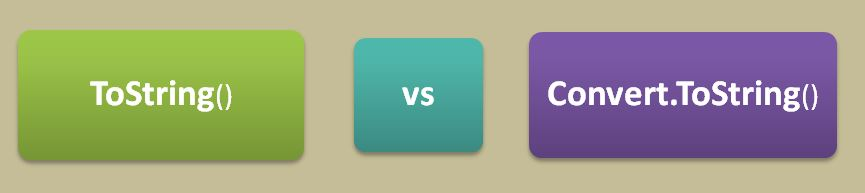#### 1. ToString() in C#

It is defined on every object in C#. It is used to convert the current instance of an object to its string representation.

Points to Remember

1. If the value being converted to a string is null, ToString() will throw a NullReferenceException
2. ToString() is a virtual method, which means that it can be overridden by derived classes to provide a custom implementation.
3. ToString() can only be called on an object instance. In C#, an object instance is a specific occurrence of a class or a struct that has been created in memory at runtime.

Point 1 - If the value being converted to a string is null, ToString() will throw a NullReferenceException.

Here is an example.

``````using System;
using System.Text;

namespace FirstProgram
{
class Program
{
static void Main(string[] args)
{
Employee objemp = null;
objemp.ToString();  // Will show error NullReferenceException
}
}
public class Employee
{
public string FirstName { get; set; }
public string LastName { get; set; }
}
}``````

Now run the above code will show a NullReferenceException error.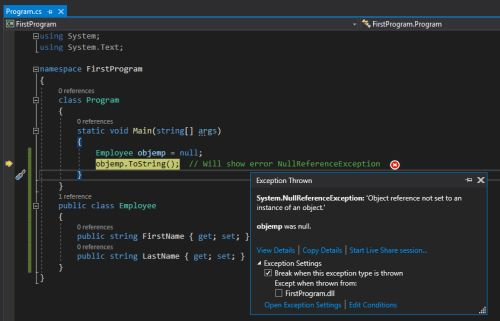Point 2 - ToString() is a virtual method, which means that it can be overridden by derived classes to provide a custom implementation.

The following code creates a objemp object and calls its ToString() method. Here is an example.

``````using System;
using System.Text;

namespace FirstProgram
{
class Program
{
static void Main(string[] args)
{
Employee objemp = new Employee { FirstName = "Rohatash", LastName = "25" };
string personString = objemp.ToString();
Console.WriteLine(personString); // Output: Name: Rohatash, Age: 25
}
}
public class Employee
{
public string FirstName { get; set; }
public string LastName { get; set; }

public override string ToString()
{
return \$"FirstName: {FirstName}, LastName: {LastName}";
}
}
}``````

Now run the above code.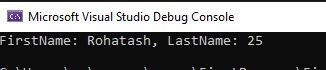Point 3 - ToString() can only be called on an object instance. In C#, an object instance is a specific occurrence of a class or a struct that has been created in memory at runtime.

``````using System;
using System.Text;

namespace FirstProgram
{
class Program
{
static void Main(string[] args)
{
Employee objemp = new Employee();   // This creates a new instance of the Employee class in memory,
}
}
public class Employee
{
public string FirstName { get; set; }
public string LastName { get; set; }
}
}``````

In the above code that line creates a new instance of the Employee class in memory. ToString() can be called on that object instance.

``Employee objemp = new Employee(); // This creates a new instance of the Employee class in memory, ``

#### 2. Convert.ToString() in C#

It is defined in the System.Convert class. It is used to convert a value to its string representation.

Points to Remember

1. If the value being converted to a string is null, Convert.ToString() will return an empty string.
2. It is a static method and cannot be overridden.
3. It can be called on a value type directly.

Point 1 - If the value being converted to a string is null, Convert.ToString() will return an empty string.

``````using System;
using System.Text;

namespace FirstProgram
{
class Program
{
static void Main(string[] args)
{
Employee objemp = null;
Console.WriteLine("Value of Object :" + Convert.ToString(objemp));
}
}
public class Employee
{
public string FirstName { get; set; }
public string LastName { get; set; }
}
}``````

Now run the above code will return an empty string.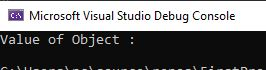Point 2 - It is a static method, which means that it can not be overridden.

The following code creates a objemp object and calls its ToString() method. Here is an example.

``````int x = 567;
string xString = Convert.ToString(x); // This is valid
Convert converter = new Convert();
string xString = converter.ToString(x); // This is invalid and will result in a compile-time error``````

In the above example we cannot create an instance of the `Convert` class and call ToString() on that instance, as shown in the second line of the code. This will result in a compile-time error, because ToString() is a static method that can only be called on the Convert class itself, not on an instance of the class.

Point 3 - It can be called on a value type directly.

The following code called Convert.ToString() method on a value type directly. Here is an example.

``````using System;
using System.Text;

namespace FirstProgram
{
class Program
{
static void Main(string[] args)
{
int x = 567;
string mystring = Convert.ToString(x); // This will return "567"
Console.WriteLine(mystring);
}
}
}``````

Now run the above code will return 567.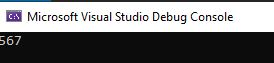#### Difference between ToString() and Convert.ToString() Method in C#

S.No. ToString() Convert.ToString()
1 If the value being converted to a string is null, ToString() will throw a NullReferenceException If the value being converted to a string is null, Convert.ToString() will return an empty string.
2 ToString() is a virtual method, which means that it can be overridden by derived classes It is a static method and cannot be overridden.
3 In C#, an object instance is a specific occurrence of a class or a struct that has been created in memory at runtime. It can be called on a value type directly.

Prev Next